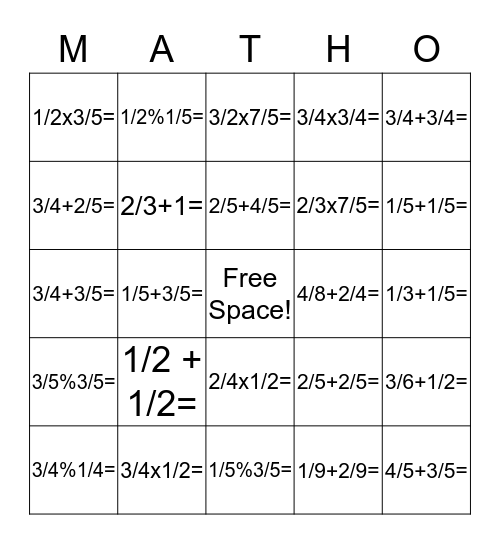# Fraction Matho !This bingo card has a free space and 24 words: 1/2 + 1/2=, 3/4x1/2=, 2/5+4/5=, 3/4%1/4=, 3/4+3/4=, 1/2x3/5=, 3/4+2/5=, 1/2%1/5=, 4/8+2/4=, 2/4x1/2=, 2/3x7/5=, 3/4+3/5=, 2/5+2/5=, 3/6+1/2=, 2/3+1=, 1/9+2/9=, 1/3+1/5=, 3/2x7/5=, 1/5+1/5=, 1/5+3/5=, 4/5+3/5=, 3/5%3/5=, 3/4x3/4= and 1/5%3/5=.

## Play Online